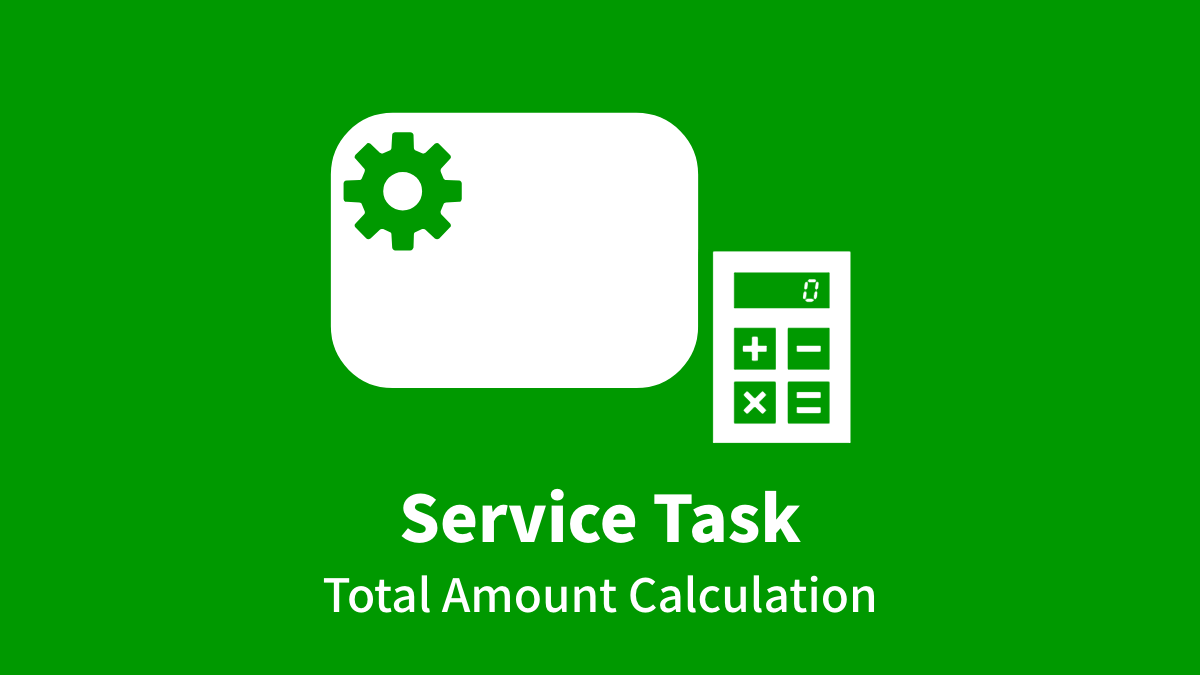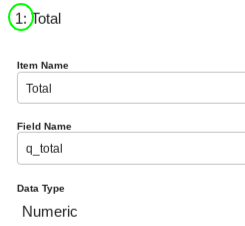I’m wondering whether you would like to add an automatic money calculation function to the workflow App for goods purchasing requests. The App will allow you to calculate not only the total and subtotal, but also to change the formula depending on the conditions.

In this post, I would like to illustrate how to implement a system that not only calculates the total amount based on the purchase list but also does a discount calculation which deducts 50% from payments exceeding \$1000 of the total amount using a split in the workflow diagram. A Table-type Data Item and a Service Task (Data Assignment) are vital elements for the design of this App.

## Calculation of the Total Amount with a Table-type Data Item

First of all, we’d like you to create an App, setting a Table-type Data Item for listing the purchased items and make it calculate the total automatically.

### Workflow Diagram

Please create a new App and set up the workflow diagram as follows:

### Data Items

Let’s prepare the following data items.

#### Purchase List Settings

In the Purchase List (Table-type Data Item) you should add 4 table columns, which are labelled Description, Unit Price, Quantity, and Subtotal respectively.

In the Subtotal column the subtotal of each row in the table is calculated and saved. Please remember to check the box “Show total value at the bottom of list” as well.

Then you should set up a Numeric-type Data Item that saves the total value of the Table-type Data Item using the arithmetic expression settings window. The expression is automatically inserted into the text box.

#### Data editing permission Settings

Please set up as in the following figure.

You’ve created an app that calculates the total amount from the Purchase list using a Table-type Data Item. Please exit editing and release it now to see how it works.

When you finish the Task after entering the unit price and quantity of an item in the Purchase list, the total amount will be saved in the Total section.

## Discount Calculations Using Service Task (Data Assignment)

Now let’s implement a workflow that performs automatic calculations under the condition of a 50% discount for payments where the total is over \$1000.

### Workflow Diagram

Let’s add some changes to the initial workflow diagram that you made as follows:

Set a Service Task (Data Assignment) in each split flow where the condition of the Task is set to more than \$1000 and less than \$1000 in the total amount. That enables the token to advance to the Task and set the condition of a 50% discount for payments exceeding \$1000 in the total amount.

#### Settings in Exclusive Gateway (XOR)

You use an Exclusive Gateway (XOR) to control the flow based on whether the total amount is over \$1000.

You have to add a flow for cases of over \$1000 and set its destination to “Discount”, whereas you set the destination of the Default Flow to “No Discount”.

For the condition of the Total which is set over \$1000, the Conditional Expression Setting is set as follows.

This setting enables the token to advance to the Discount Processing Task if the Total is \$1000 or more, whereas the token advances to the No Discount Task if the total is less than \$1000.

### Data Item Settings

Please add a Numeric-type Data Item called “Total Discounted Amount” to save the discounted total.

### Settings in Service Task (Data Assignment)

Let’s set up two Service Tasks (Data Assignment) respectively for the No Discount and Discount branches.

#### No Discount

Set up an expression for the Total which is less than \$1000. Since it will not apply any discount, the value of Total should be saved in the Total Discounted Amount data item.

#### Discount

Set up an expression for the Total which is over \$1000. A 50% discount is given to the amount over \$1000 so the expression is set as follows:

``1000 + (#q_total - 1000) * 0.5``

* For the Numeric-type, numbers below the range of decimal digits specified in the settings of the Data item are removed (e.g. if the range of decimal digits is 0, then the result of 1.5 (3 * 0.5) becomes 1 due to removing the first decimal place). The same thing will perform in the Numeric-type item in the table columns.

The settings of the App are now complete. You have to exit editing and release it to see how it works. In particular, I would like you to check that splitting by the Total is working and check for any mistakes in discount calculations.

### Use of an Arithmetic expression in the Settings window (Numeric-type Data Item)

This time I used a Service Task (Data Assignment) for the discount calculations to cope with the splitting process. However, the Expression field in the settings window of a Numeric-type Data Item can be used for arithmetic operations as well. For example, you can achieve a 50% discount by setting the same expression as with the Discount Task like in the figure below. However, you should remember that the Expression field in the settings window can’t set a split condition for a 50% discount for payments over \$1000.

You cannot embed data with a field name (e.g. #q _ total) in the arithmetic expression of the Numeric-type Data Item’s settings window. Please write data [‘ 1 ’] using the Data Item number, which is located in the Edit screen’s Data Item tab and the upper left corner of each settings window..

Although within the settings window of a Numeric-type Data Item it is not possible to change expressions according to any conditions, it may not be necessary if the App doesn’t require a splitting process. Please use the property according to the implementation you want.

As explained above, the use of a Table-type Data Item and Service Task (Data Assignment) will make it possible to perform various calculations. Questetra BPM Suite will help with troublesome monetary calculations that can change the formula according to the conditions faster without bothering any staff members.

### 3 thoughts on “Let’s Calculate the Total Amount Automatically”

1. Pingback: Numeric-Type – Questetra Support

2. Pingback: Table-Type – Questetra Support